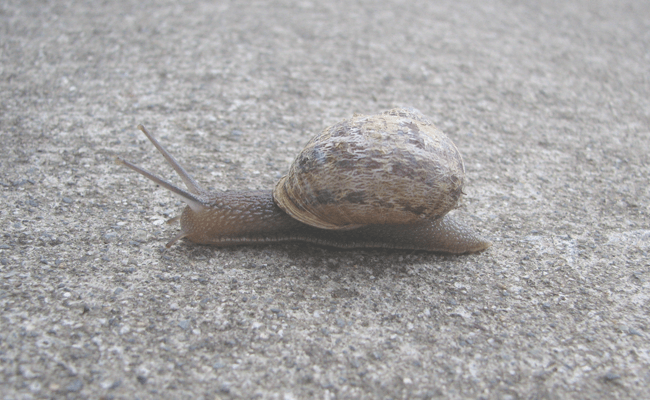# How to Calculate Delay Time for Beat SynchronizationCalculating the delay time for beat synchronization is often a point of confusion. But it don’t have to be. In essence, it is just a simple mathematical formula that you can deduce easily if you once understood it fully. In this article, I give you a brief introduction to delay as a plugin for production and a step by step process on how to calculate the delay time for beat sync.

## What is Delay?

The simplest form of a delay is a copy of a signal that is delayed in time. As a tool in music production, hardware device or software plugin, a delay has more parameters than just delay time. Normally, you have the following variables to play with:

• Feedback,Repeat; Indicates how fast the volume of the delay signal dies away
• Delay time; Mostly in ms or if beat sync in note value; Time between original signal and repetition
• Filter, High-cut, Low-cut; Influences the sound color of the repeated signal
• Dry/Wet; Volume ratio between original and delayed signal

There many delays that are more complex like the Delay Designer of Logic. You can create many interesting sound field and rhythmic patterns with it. Give it a try.

## How to Calculate Delay Time for Beat Synchronization

Nowadays, almost every delay plugin has an implemented function for beat synchronization. Still, it is very useful to understand how the delay time is calculated. It will come in handy in many situations.

First, you have to find out what the tempo of your song is. Lets say it is 100 bpm. Bpm stands for beats per minute. There are 60 seconds in a minute. Therefore, the time between two beats is 60/100 = 0.6 seconds. Multiplied by 1000 you get 600 ms (milliseconds) per beat. Surprise, you already calculated the delay time for a quarter note. If you want to calculate the delay time for an eighth note, just divide the 600 ms by 2 = 300 ms. It is as simple as that.

And now, the same in form of a list:

1. What is your songs tempo? 60, 80, 100, x bpm?
2. Divide 60 (seconds) by your tempo: For Example 60 / 80 = 0,75.
3. Multiply the number by 1000 to get the delay time in milliseconds. 0.75*1000 = 750 ms per quarter note.
4. If you want to calculate a different interval than a quarter note, multiply your result in milliseconds;
• by 0.166 (or 1/6) for a sixteenth triplet note
• by 0.25 for a sixteenth note
• by 0.333 (or 1/3) for a eighth triplet note
• by 0.5 for a eighth note
• by o.666 (or 2/3) for a quarter triplet note
• by 0.75 for a dotted eighth note
• by 1 for a quarter note
• by 1.333 for a half triplet note
• by 1.5 for a dotted quarter note
• by 2 for a half note
• by 3 for a dotted half note
• by 4 for a whole note

## Exercise

Take your favorite delay and calculate the delay time for different note values in varying tempos. Listen to them to check if you were right.